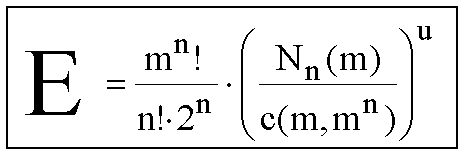Estimates of the number of magic squares, cubes, ... (hypercubes)
 E-Number of a magic hypercube Dimension: n     Order: mwith   um = mn - (m - 1)n - 1 + 2n-1   and   Nn(m) = Number of magic series.

What improvements of the simple concept on the previous page are neccessary?
- How many 'regular number hypercubes' do exist?
- How many aspects does a hypercube of dimension n posses?
- What is the probability of the first magic line?
- How many unforced magic lines are there?
Define a normal number hypercube of dimension n and order m as a mn-array of positive integers from 1 to mn.
There are mn! ways to arrange the mn numbers. Therefore we get
 W = mn! normal number hypercubes

Aspects
The number A of aspects of a hypercube depends on its dimension n but not on its order m.
a) There are 2n ways to combine reflections of a hypercube in the direction of any axis.
This can also be considered as inverting the order of certain coordinates.
b) Other aspects can be achieved by interchanging coordinates:
As we got n coordinates, there are n! permutations of them.
Thus we get the following formula for the number of aspects:
 A = n! · 2n aspects of hypercubes

Magic probability
There are Nn(m) magic series of dimension n and order m.
And there are c(m, mn) combinations of m numbers selected from mn numbers.
Thus the exact probability of magicness for a random line with m numbers and no further constraints is:
 p = Nn(m) / c(m, mn) probability of the first magic line

Unforced magic lines
Again we assume the same probability p for other lines to be magic if they are not forced to be magic.
The number u of unforced magic lines depends on the dimension, the order and the magic properties of the hypercube.
Consider a random number cube of order m and begin to check the rows on magicness. Only one of the m2 rows is forced to be magic. Now check the columns: already the nth column is forced to be magic. Because m rows and m columns form a semi-magic sub-square of the cube. That is the same in the other planes. From m pillars there is one forced to be magic for the first (m-1) planes which they build with rows. In the last plane every pillar is forced to be magic (consider planes formed by pillars and columns).
That makes   u = m2 - 1 + m · (m - 1) + (m - 1)2  unforced magic lines of an order-m semi-magic cube.
And   u = mn-1 - 1 + mn-2 · (m - 1)1 + mn-3 · (m - 1)2 + ... + (m - 1)n-1   unforced magic lines in a semi-magic hypercube.
This formula can be written in a perplexing simple way:
 usm = mn - (m - 1)n - 1 unforced magic lines in a semi-magic hypercube.
In a regular magic hypercube the longest diagonals (the so-called n-agonals) have to be magic. As these lines connect two opposite corners there are half as much n-agonals than corners of the hypercube.
 um = mn - (m - 1)n - 1 + 2n-1 unforced magic lines in a regular magic hypercube.

Definition
Consider a regular number hypercube of order m and dimension n with certain magic properties (semi-magic or normal magic or pandiagonal magic or ...) and u unforced magic lines then the E-number (estimate number) of this hypercube is defined by the following equation:
 E = mn! · pu / A with p = Nn(m) / c(m, mn) and A = n! · 2n
- Nn(m) is the number of magic series of dimension n and order m. See magic series!
- c(m, mn) is the number of combinations of m numbers selected from mn ones.
- A is the number of aspects that a hypercube has got in dimension n.
- u is the number of unforced magic lines. See chapter above.